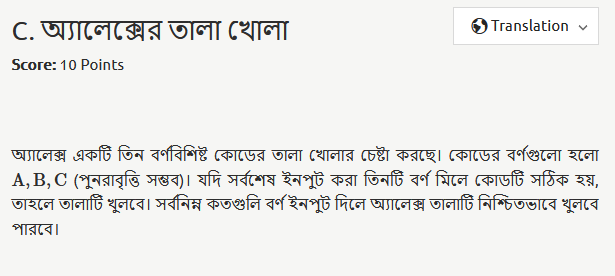# Calibration Round #7why is the ans 29? isn’t it supposed to be 27

1 Like

The answer is 27+2 = 29. No error here

1 Like

Why +2?

1 Like
Solution

For smaller case, let there be 2 letter A,B
There are 4 combination:
AA,AB,BA,BB

Now if you keep pressing the button,for best case, any 2 letter match one of the 4 combination and no combination exist more than once , then let the term the letter by 1,2,3…

For example , pressing button like this,
AABABBAAB… so here 1=A, 2=A, 3=B, …

Now , in the best case, 12 ≠ 23 ≠ 34 ≠ ...
As there are 4 combination, then the succesful code (read question) = 45.

So they need at least 4+1 = 4+(2-1)

Back to the main point,